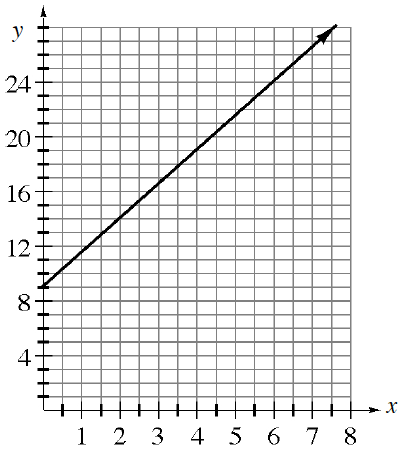### Home > CC4 > Chapter 5 > Lesson 5.3.1 > Problem5-91

5-91.This problem is a checkpoint for writing the equation of a line. It will be referred to as Checkpoint 5. Homework Help ✎

Use the given information to write the equation of each line.

1. Has a slope of $2$ and passing through $(10, 17)$.

2. Passing through $(1, –4)$ and $(–2, 5)$.

3.  $x$ $-6$ $-3$ $0$ $3$ $6$ $y$ $-6$ $-4$ $-2$ $0$ $2$
4. The line graphed below.